# Operator topology

(diff) ← Older revision | Latest revision (diff) | Newer revision → (diff)

A topology on the space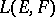of continuous linear mappings from one topological vector spaceinto another topological vector space, converting the spaceinto a topological vector space. Letbe a locally convex space and let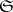be a family of bounded subsets ofsuch that the linear hull of the union of the sets of this family is dense in. Letbe a basis of neighbourhoods of zero in. The family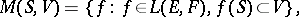whereruns throughandthrough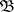, is a basis of neighbourhoods of zero for a unique topology that is invariant with respect to translation, which is an operator topology and which converts the spaceinto a locally convex space; this topology is called the-topology on.

Examples. I) Let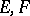be locally convex spaces. 1) Letbe the family of all finite subsets in; the corresponding-topology (on) is called the topology of simple (or pointwise) convergence. 2) Letbe the family of all convex balanced compact subsets of; the corresponding topology is called the topology of convex balanced compact convergence. 3) Letbe the family of all pre-compact subsets of; the corresponding-topology is called the topology of pre-compact convergence. 4) Letbe the family of all bounded subsets; the corresponding topology is called the topology of bounded convergence.

II) Ifare Banach spaces considered simultaneously in the weak or strong (norm) topology, then the corresponding spacescoincide algebraically; the corresponding topologies of simple convergence are called the weak or strong operator topologies on. The strong operator topology majorizes the weak operator topology; both are compatible with the duality betweenand the space of functionals onof the form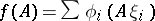, where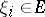,,.

III) Letbe Hilbert spaces and letbe countable direct sums of the Hilbert spaces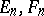, respectively, where,for all integer; letbe the imbedding of the spaceinto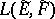defined by the condition that for any operator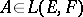the restriction of the operator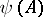to the subspace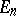mapsinto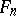and coincides onwith the operator. Then the complete pre-image inof the weak (strong) operator topology onis called the ultra-weak (correspondingly, ultra-strong) operator topology on. The ultra-weak (ultra-strong) topology majorizes the weak (strong) operator topology. A symmetric subalgebraof the algebra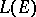of all bounded linear operators on a Hilbert space, containing the identity operator, coincides with the set of all operators fromthat commute with each operator fromthat commutes with all operators from, if and only ifis closed in the weak (or strong, or ultra-weak, or ultra-strong) operator topology, i.e. is a von Neumann algebra.

How to Cite This Entry:
Operator topology. Encyclopedia of Mathematics. URL: http://encyclopediaofmath.org/index.php?title=Operator_topology&oldid=12370
This article was adapted from an original article by A.I. Shtern (originator), which appeared in Encyclopedia of Mathematics - ISBN 1402006098. See original article